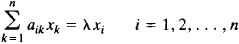# eigenvector

Also found in: Dictionary, Acronyms, Wikipedia.

## eigenvector

[′ī·gən‚vek·tər]
(mathematics)
A nonzero vector v whose direction is not changed by a given linear transformation T ; that is, T (v) = λ v for some scalar λ. Also known as characteristic vector.
McGraw-Hill Dictionary of Scientific & Technical Terms, 6E, Copyright © 2003 by The McGraw-Hill Companies, Inc.
The following article is from The Great Soviet Encyclopedia (1979). It might be outdated or ideologically biased.

## Eigenvector

(or characteristic vector). An eigenvector of a linear transformation is a vector that does not change direction under the transformation and is simply multiplied by a scalar. For example, the eigenvectors of a transformation composed of rotations about some axis and of contraction toward the plane perpendicular to the axis are vectors directed along the axis.

The coordinates x1x2,..., xn of the eigenvectors of a transformation of n-dimensional space with the matrix ║aik║ satisfy the system of homogeneous linear equationswhere λ is an eigenvalue of the matrix. If the matrix of a transformation is Hermitian, then the eigenvectors are mutually perpendicular. As a result of a Hermitian transformation, a sphere becomes an ellipsoid whose major axes are eigenvectors of the transformation.

## eigenvector

(mathematics)
A vector which, when acted on by a particular linear transformation, produces a scalar multiple of the original vector. The scalar in question is called the eigenvalue corresponding to this eigenvector.

It should be noted that "vector" here means "element of a vector space" which can include many mathematical entities. Ordinary vectors are elements of a vector space, and multiplication by a matrix is a linear transformation on them; smooth functions "are vectors", and many partial differential operators are linear transformations on the space of such functions; quantum-mechanical states "are vectors", and observables are linear transformations on the state space.

An important theorem says, roughly, that certain linear transformations have enough eigenvectors that they form a basis of the whole vector states. This is why Fourier analysis works, and why in quantum mechanics every state is a superposition of eigenstates of observables.

An eigenvector is a (representative member of a) fixed point of the map on the projective plane induced by a linear map.